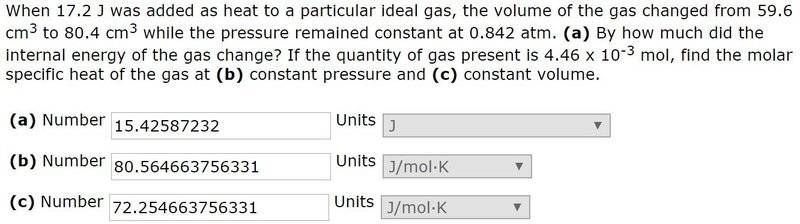# Interpreting Molar Specific Heat number

## Homework StatementCv=(f/2)R

## The Attempt at a Solution

I have no problem getting the right answers. My question is this: If Cv=72.254 and if Cv = (f/2)R, that implies that f = 17.381. I understand that f represents the gas particle's degrees of freedom. How does this particle have 17.381 degrees of freedom?

Obviously I'm missing something fundamental here, and look forward to your gentle and enlightening response![/B]

#### Attachments

How does this particle have 17.381 degrees of freedom?
Although the numbers in the problem may just be made up, a gas could theoretically have 18 degrees of freedom if its molecules contained six atoms. The number of transnational and rotational degrees of freedom is ##5## for linear molecules and ##6## for non-linear molecules. The number of vibrational degrees of freedom is ##3N-5## for a ##N## atom linear molecule and ##3N-6## for a non-linear molecule. The total number of possible degrees of freedom is then ##3N## for all molecules.

One issue however, is that the vibrational modes are harder to excite than the translational and rotational modes. This means that if the temperature of the gas is not sufficiently high, only some of the molecules will have enough energy to explore all of the possible modes. This means that the energy is not evenly divided among all the modes as the equal partition theorem would tell you. This may lead to a non-integer number for the degrees of freedom if you try to calculate it using data at low or room temperatures.

•Bystander
Thank you so much for your thoughtful response - this is very enlightening. I'll definitely investigate vibration modes further. I'm not, though, quite sure I'm following your statement (below). A multi-atom molecule has 3N-5 dof if it's linear (translation?), and 3N-6 if it's a non-linear molecule (oscillatory?) if temperature is sufficiently high. Where, then, does "the total number of possible degrees of freedom is then 3N for all molecules" come from, or what does it refer to?

Thank you again!

The number of transnational and rotational degrees of freedom is ##5## for linear molecules and ##6## for non-linear molecules. The number of vibrational degrees of freedom is ##3N-5## for a ##N## atom linear molecule and ##3N-6## for a non-linear molecule. The total number of possible degrees of freedom is then ##3N## for all molecules.

DrClaude
Mentor
A multi-atom molecule has 3N-5 dof if it's linear (translation?), and 3N-6 if it's a non-linear molecule (oscillatory?) if temperature is sufficiently high.
These equations represent the number of vibrational degrees of freedom.

Where, then, does "the total number of possible degrees of freedom is then 3N for all molecules" come from, or what does it refer to?
That's because space is 3D: you need three coordinates to specify the position of each atom. The total number of degrees of freedom is thus ##3N##. You can then count 3 degrees of freedom to position the centre-of-mass of the molecule, and 3 dof for rotation (e.g., the 3 Euler angles). That leaves ##3N-6## degrees of freedom for the relative position of the atoms with respect to one-another, hence for vibrations. In the case of a linear molecules, only two angles are needed to position it in space, so ##3N-5## are left for vibrations.

•NFuller
These equations represent the number of vibrational degrees of freedom.

That's because space is 3D: you need three coordinates to specify the position of each atom. The total number of degrees of freedom is thus ##3N##. You can then count 3 degrees of freedom to position the centre-of-mass of the molecule, and 3 dof for rotation (e.g., the 3 Euler angles). That leaves ##3N-6## degrees of freedom for the relative position of the atoms with respect to one-another, hence for vibrations. In the case of a linear molecules, only two angles are needed to position it in space, so ##3N-5## are left for vibrations.
Ah - thank you so much, got it!!